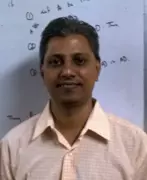X
Infinite matrices and their recent applications
Published in Springer International Publishing
2016
Pages: 1 - 118
Abstract
This monograph covers the theory of finite and infinite matrices over the fields of real numbers, complex numbers and over quaternions. Emphasizing topics such as sections or truncations and their relationship to the linear operator theory on certain specific separable and sequence spaces, the authors explore techniques like conformal mapping, iterations and truncations that are used to derive precise estimates in some cases and explicit lower and upper bounds for solutions in the other cases. Most of the matrices considered in this monograph have typically special structures like being diagonally dominated or tridiagonal, possess certain sign distributions and are frequently nonsingular. Such matrices arise, for instance, from solution methods for elliptic partial differential equations. The authors focus on both theoretical and computational aspects concerning infinite linear algebraic equations, differential systems and infinite linear programming, among others. Additionally, the authors cover topics such as Bessel’s and Mathieu’s equations, viscous fluid flow in doubly connected regions, digital circuit dynamics and eigenvalues of the Laplacian © Springer International Publishing Switzerland 2016.
Journal Data powered by TypesetInfinite Matrices and Their Recent Applications Data powered by TypesetSpringer International Publishing No
Authors (1)
•Concepts (17)
•Computation theory
•Conformal mapping
•Eigenvalues and eigenfunctions
•Flow of fluids
•Linear equations
•Linear programming
•Mathematical operators
•Partial differential equations
•Computational aspects
•DIFFERENTIAL SYSTEMS
•DOUBLY CONNECTED REGIONS
•ELLIPTIC PARTIAL DIFFERENTIAL EQUATION
•LINEAR ALGEBRAIC EQUATION
•LINEAR OPERATOR THEORIES
•Lower and upper bounds
•VISCOUS FLUID FLOW
•Matrix algebra